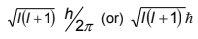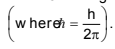# Types of Quantum Numbers

1.  Principal quantum number (n):

It tells the main shell in which the electron resides and the approximate distance of the electron from the nucleus. It also tells the maximum number of electrons a shell can accommodate is 2n2, where n is the principal quantum number.

Shell                                           K L M N

Principal quantum number (n)    1 2 3 4

Maximum number of electrons   2 8 18 32

2. Azimuthal or angular momentum quantum number (l):

This represents the number of subshells present in the main shell. These subsidiary orbits within a shell will be denoted as 1,2,3,4,... or s,p,d,f... This tells the shape of the subshells. The orbital angular momentum of the electron is given asfor a particular value of ‘n’For a given value of n values of possible l vary from 0 to n – 1.

3. The magnetic quantum number (m):

An electron due to its angular motion around the nucleus generates an electric field. This electric field is expected to produce a magnetic field. Under the influence of external magnetic field, the electrons of a subshell can orient themselves in certain preferred regions of space around the nucleus called orbitals.

The magnetic quantum number determines the number of preferred orientations of the electron present in a subshell. The values allowed depends on the value of l, the angular momentum quantum number, m can assume all integral values between –l to +l including zero. Thus m can be –1, 0, +1 for l = 1. Total values of m associated with a particular value of l is given by 2l + 1.

4. The spin quantum number (s):

Just like earth not only revolves around the sun but also spins about its own axis, an electron in an atom not only revolves around the nucleus but also spins about its own axis.

Since an electron can spin either in clockwise direction or in anticlockwise direction, therefore, for any particular value of magnetic quantum number, spin quantum number can have two values, i.e., +1/2 and –1/2 or these are represented by two arrows pointing in the opposite directions, i.e.,  and .

When an electron goes to a vacant orbital, it can have a clockwise or anti clockwise spin i.e., +1/2 or –1/2. This quantum number helps to explain the magnetic properties of the substances.

Post By : Mamta Singh 09 Jan, 2020 2204 views Chemistry